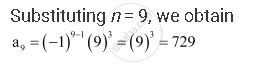CBSE (Arts) Class 11CBSE
Share
Notifications

View all notifications
Books Shortlist
Your shortlist is empty

# Find The 9th Term in the Following Sequence Whose Nth Term is A_N = (–1)^(N – 1) N^3; A_9 - CBSE (Arts) Class 11 - Mathematics

Login
Create free account

Forgot password?
ConceptConcept of Sequences

#### Question

Find the 9th term in the following sequence whose nth term is a_n = (–1)^(n – 1) n^3; a_9

#### SolutionIs there an error in this question or solution?

#### APPEARS IN

NCERT Solution for Mathematics Textbook for Class 11 (2018 to Current)
Chapter 9: Sequences and Series
Q: 9 | Page no. 180
Solution Find The 9th Term in the Following Sequence Whose Nth Term is A_N = (–1)^(N – 1) N^3; A_9 Concept: Concept of Sequences.
S